# Percentages

Examples of percentages are all around us. You might particularly see percentages used in the supermarket, or in a shop when a sale is on.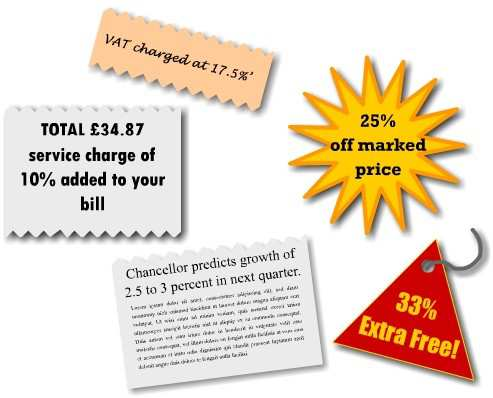### What is a percentage?

‘Per cent’ literally means ‘out of each hundred’. So if we could divide up the whole into 100 parts, 10% would represent 10 out of the 100 parts, 25% would be 25 parts, and 50%.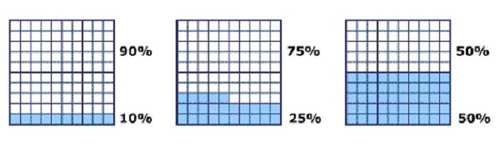Notice that taking 50% is just the same as taking half of something. It is also true that taking 25% is like taking a quarter, 20% is a fifth, and 10% is a tenth.

#### Example 1

Mortgages are advertised at the following rates. Which of these banks/building societies give the best rate?

 Alliance & Chester 12.5% Royal Bank of Wales 5.5% Alternative Life 8.3% Southern Rock 23.7% Lancashire Building Society 7.6% Uncooperative Bank 19.2%
Solution

The Royal Bank of Wales gives the lowest interest rate, and so this would be the best choice.

#### Example 2

On a carton of Tropical Fruit Juice, the carton states that the contents are as given below. What is wrong with this statement?

Orange Juice Pineapple Juice Mango Extract Papaya Extract
40% 30% 25% 15%
Solution

These percentage values should add up to 100%, but they add up to 110%, which is not possible.

#### Example 3

The shoe shop in the high street is closing down, so I pop in to see if I can find a bargain. All shoes are being sold at 50% off the marked price. I find a really nice pair of shoes priced at £60. How much would it cost to treat myself to this pair of shoes?

Solution

The original price can be thought of as the full 100%, and we need to find the discount price.

So, how much is the price reduced by? The discount is 50%, or 50 parts out of 100 of the original price. Where we read the word of we can replace this with multiplied by, and write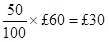So the shoes are being sold at £30 off the original price, which leaves £30 to pay.

You may have known straight away that ‘50% off’ is the same as saying ‘half price’, and you’d have been able to work out immediately that half of £60 is £30.

### Finding a percentage

To know the real value of a percentage, we need to know what it is a percentage of. For instance, in our shoe shop in Example 3, all shoes are 50% off. For a £60 pair of shoes this means £30 off, but if I had chosen a £20 pair of shoes the reduction would be only £10.

#### Example 4

The pass mark for an exam is 60%, and there are 40 questions on the exam sheet. How many questions do I need to get right in order to pass the exam?

Solution

If I got all the questions correct, I would score 100%. Fortunately, I only need to get 60%, or 60 out of every hundred of the questions correct. There are only 40 questions, so let’s take sixty hundredths of these 40 questions.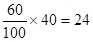So 24 out of 40 questions corresponds to 60%, and as long as I can answer at least 24 questions correctly I will pass the exam.

### Expressing one quantity as a percentage of another

To express one quantity as a percentage of another, we must first express this as a proportion, and then convert this to a percentage by multiplying by 100.

#### Example 5

In an exam I managed to answer 18 questions correctly out of a total of 30. What is my score as a percentage?

Solution

In this question we are being asked to convert to a percentage. If 100% represents the whole, or in this case, the total number of questions, we are asking how many parts out of 100 would we have? In other words, how many parts out of 100 does 18 out of 30 correspond to?

To do this, we take our proportion and times by 100So I have scored 60% on this exam.

### Exercise 1

My tin of Apples and Pears is 35% apple and 40% syrup. What percentage of it is pear?

#### Solution

The contents of the tin must add up to 100%. The apple and syrup add up to 75%, so the remaining 25% must be pear.

### Exercise 2

The brand of teabags I normally buy have a special offer on. The offer is 25% Extra Free. I normally buy a packet of 48 teabags. How many teabags will I get in my packet this time?

#### Solution

I will get an extra 25% of 48 teabags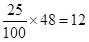This means an extra 12 teabags. Adding this to the 48 teabags in a normal packet, I will get a total of 60 teabags.

Tip:

A quicker way of doing this calculation would be to find 125% of 48.This would include a whole packet of teabags (100%) plus the extra 25%.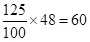By doing it this way, we have reduced our 2 steps into just one.

### Exercise 3

The pass mark for an exam is 40%, and there are 25 questions on the exam sheet. How many questions do I need to get right in order to pass the exam?

#### Solution

I need to get at least 40%, or 40 out of every hundred, of the 25 questions correct.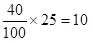So I need to answer at least 10 of the questions correctly.

### Exercise 4

Radio alarm clocks were selling at £25 but have been reduced to £20. What percentage reduction does this represent on the original price?

#### Solution

The price has been reduced by £5. As a percentage of the original price, this is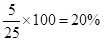So the alarm clocks are being sold at 20% off.

### Exercise 5

Which is the greatest, 25% of 32 or 20% of 35 or 15% of 40?

#### Solution

25 / 100 × 32 = 8

20 / 100 × 35 = 7

15 / 100 × 40 = 6

So 25% of 32 is the greatest.

### Exercise 6

I have £5,000 in a building society account with an interest rate of 4.1% per annum. How much interest will I earn at the end of the year?

#### Solution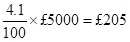### Exercise 7

Over the course of a year Jake gets the following marks in his exams. Which is Jake’s best subject?

Accounting & Finance Algebra Trigonometry Calculus
47 out of 80 39 out of 70 32 out of 40 41 out of 50

#### Solution

Let’s convert each of Jake’s marks to a percentage so that we can compare his performance in each subject.

Accounting & Finance         47 / 80 × 100 = 58.75%

Algebra                                    28 / 70 × 100 = 40%

Trigonometry                         32 / 40 × 100 = 80%

Calculus                                  36 / 50 × 100 = 72%

Jake’s highest mark was in Trigonometry, and lowest in Algebra.

### Exercise 8

Tickets for the Comedy Club cost £12 on the door. There is a 15% discount when booking in advance over the internet. How much will my ticket cost if I book on-line?

#### Solution

The discount will be

15 / 100 × £12 = £1.80

So the price to pay is £12 - £1.80, which is £10.20.

Tip:

The way to perform this calculation in a single step would be to find 85% of £12, i.e. 100% minus 15% of the price.

85 / 100 × £12 = £10.20